# LearnDash Quiz Import/Export - Overview

## Installation:

• Install the LearnDash plugin & activate it.
• Install LearnDash quiz import/export addon and activate it.
• A new “Quiz Import/ Export ”menu will appear under the LearnDash LMS menu.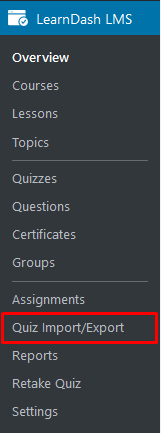Note: It is strongly recommended that you check with your hosting provider to do these server configurations changes before you try to import quizzes.

post_max_size = 48M

memory_limit = 256M

max_execution_time = 600

max_input_vars = 1000

max_input_time = 400

# How To Export Quizzes:

## Single Quiz:

When the Learndash Quiz Import/Export add-on is active on your site, a new Export button will appear in the quizzes table, under the “Quiz Export” column. To export the quiz, simply click on the export button. The quiz will be downloaded as a .xls or xlsx file, which can be imported to another site using this add-on.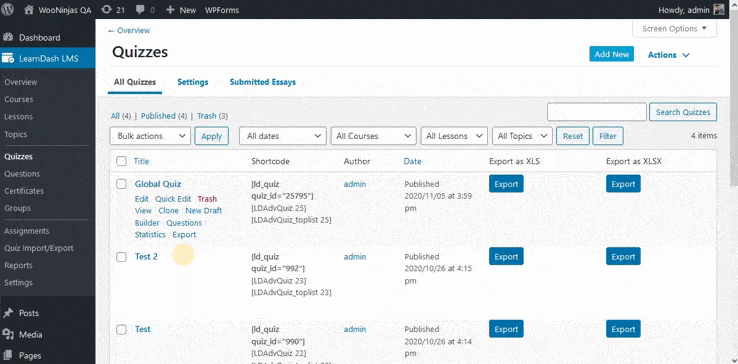## Multiple Quizzes:

When the Learndash Quiz Import/Export add-on is active on your site, you can export multiple quizzes in a single file. To export multiple quizzes at once, simply put a check on the quizzes you want to export, and from the “Bulk Options” WordPress dropdown, select. “ Export to xls and “Export to xlsx ” and click on apply

The quiz will be downloaded as a .xls or xlsx file, which can be imported to another site using this add-on.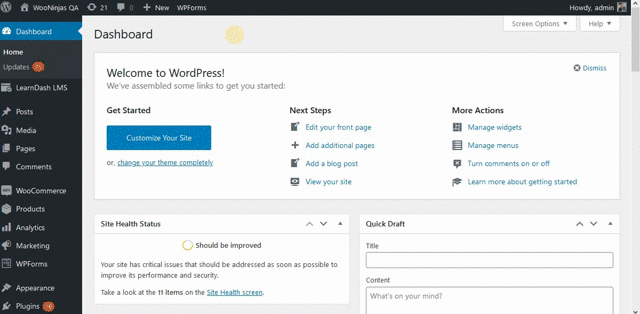# How To Import A Quiz:

To import a quiz, go to the “Import Quiz” tab from the Quiz Import/Export menu located under the main LearnDash LMS plugin menu. Here, you will find the input area for the quiz file to be imported. To complete the import, select the Microsoft Excel .xls or .xlsx file and click on the import quiz button.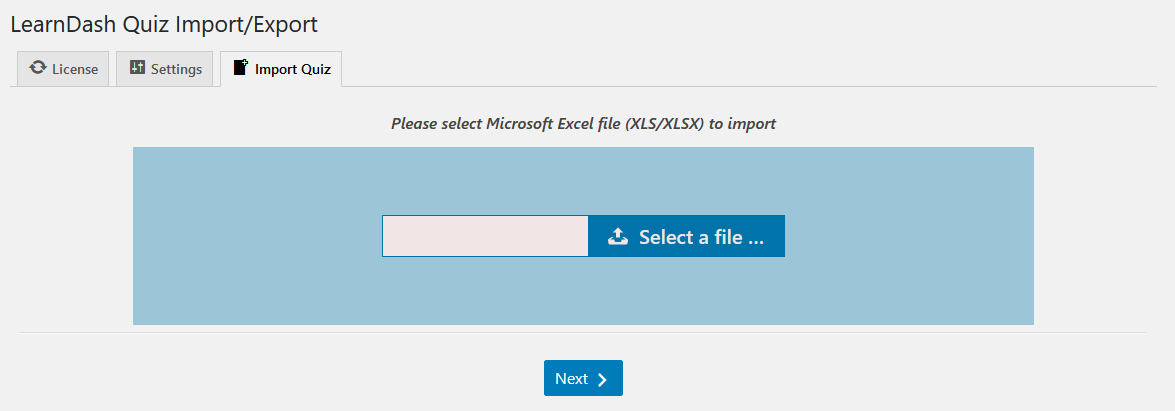# You can import several quizzes at once by adding a different quiz on each separate sheet of your spreadsheet file. Make sure to specify the individual Title for each quiz on each file.  Then, to import all created quizzes, go to the “Import Quiz” tab from the Quiz Import/Export menu located under the main LearnDash LMS plugin menu. Here, select the Microsoft Excel .xls or .xlsx file containing multiple quizzes in separate sheets and click on the import quiz button. After importing the file, you will see all imported quizzes listed on your screen, as seen in the following image:From here, you can configure the corresponding course, lesson/topic, and certificate as well as passing percentage for each of the imported quizzes.

Insert your license key in the field and then click on Activate.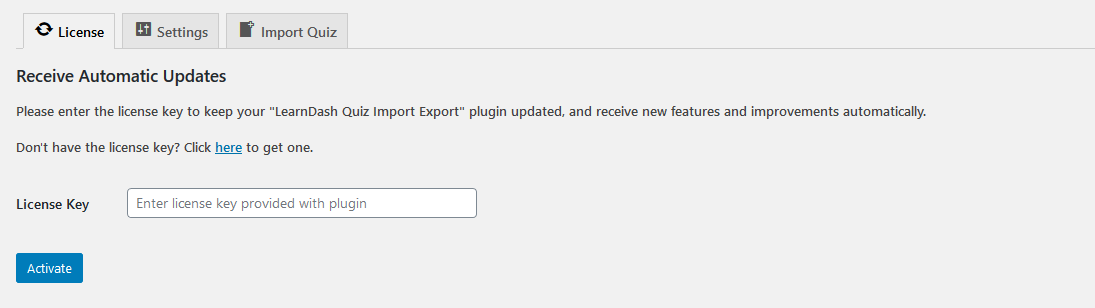# Learndash Quiz Import/Export Settings:

To configure the add-on's settings, go to the Settings tab from the main Quiz Import/Export menu under the main LearnDash LMS plugin menu. All the default LearnDash quiz options are listed here. Any new imported quiz will adopt these quiz settings.

### Apply quiz settings from:

This dropdown menu shows the existing quizzes on your website. Selecting a particular quiz from this dropdown will allow the admin to choose the specific quiz whose settings will be replicated and applied to all newly imported quizzes. You can keep this field blank if you want to import quizzes with their default settings.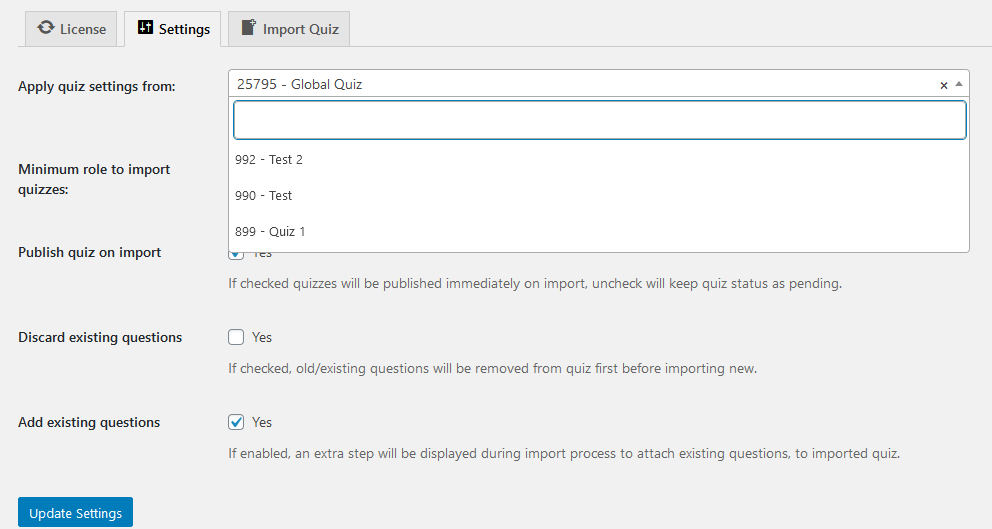### Minimum Role To Import Quizzes

This dropdown menu allows the admin to select what type of user role can access and use this plugin. If you want any author or editor to be able to import and export quizzes, you should select the respective role.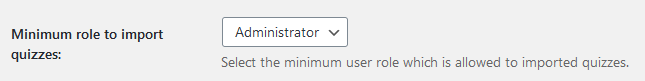### Publish quiz on import?

When this option is enabled, the quizzes will be published as soon as they are imported; disabling this option will keep quiz status as pending.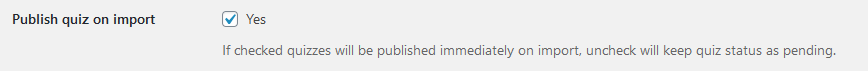### Remove existing Questions

• No: With this option selected, previously imported questions from the same quiz will not be discarded.

E.g. If you import a quiz, let’s call it. Final Quiz, with questions A, B, C, and D, and you want to import the additional questions  E, F, and G into the same quiz, If the “Remove existing Questions” option is selected to ‘No’, then all questions will be imported, including pre-existing questions, i.e., A, B, C, D, E, F, and G.

• Remove from quiz only: With this option enabled, previously imported questions from the same quiz will be discarded from the quiz. This helps prevent previously imported questions from being repeated again and again in the same quiz.

For E.g. If you import a quiz, let’s call it. Final Quiz, with questions A, B, C, and D, and you want to import the additional questions  E, F, and G into the same quiz, with the “Remove existing Questions” option selected to ‘Remove from quiz only’, after all the questions are imported, the old questions, i.e., A, B, C, and D will be discarded, and only new questions, i.e., E, F, and G will be added.

• Remove from quiz and database: With this option enabled, previously imported questions from the same quiz will be discarded from the quiz and deleted from the database. This helps prevent previously imported questions from being repeated again and again in the same quiz and questions’ database.

For E.g. If you import a quiz, let’s call it. Final Quiz, with questions A, B, C, and D, and you want to import the additional questions  E, F, and G into the same quiz, with the “Remove existing Questions” option selected to ‘Remove from quiz and database’, after all the questions are imported, the old questions, i.e., A, B, C, and D will be discarded and deleted from the questions’ database, and only new questions, i.e., E, F, and G will be added.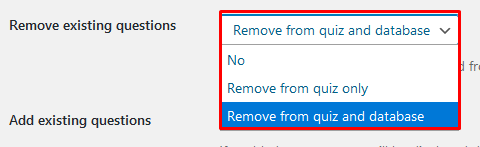### Update duplicate questions

With this option enabled, you can add astep to the import process that allows you to select any existing questions and attach them to the imported quiz in the Excel file.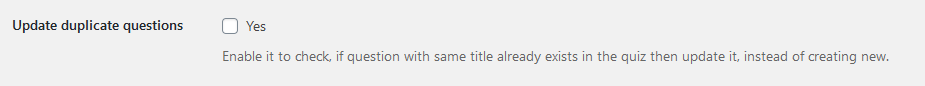With this option enabled, you can add an additional step to the import process that allows you to select which existing questions you want to attach to the imported quiz.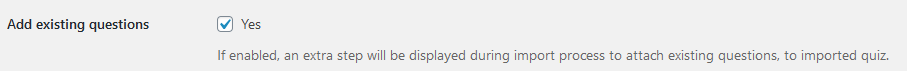There will be a dropdown menu after the upload of the quiz import file that will display all the unassociated questions so that they can be associated with the new quiz while importing.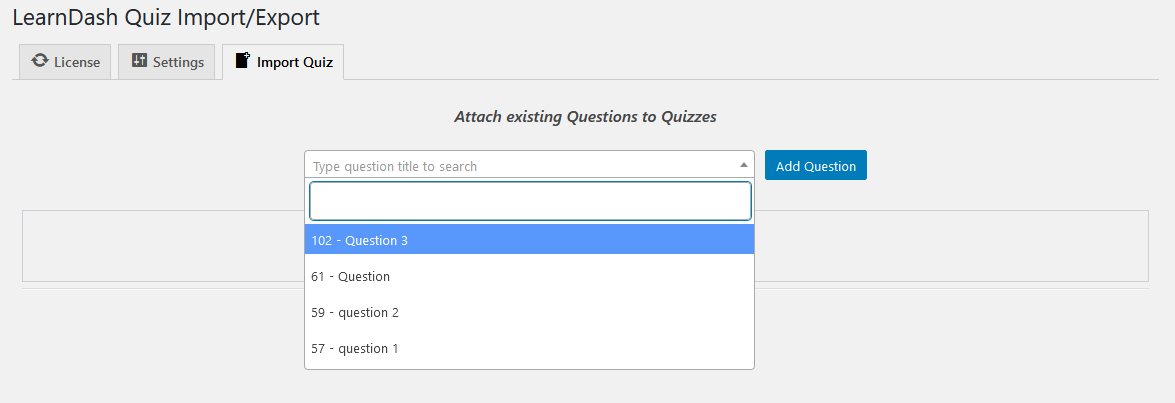### Enable Debug Logs

When this option is enabled, the debug logs are generated for easy troubleshooting by WooNinjas support teams.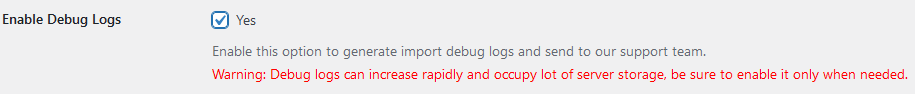Enabling this option will add another tab in LearnDash Quiz Import/Export settings which will list the logs and the name of the log file where the logs are stored, located in the wp-content/uploads/ directory.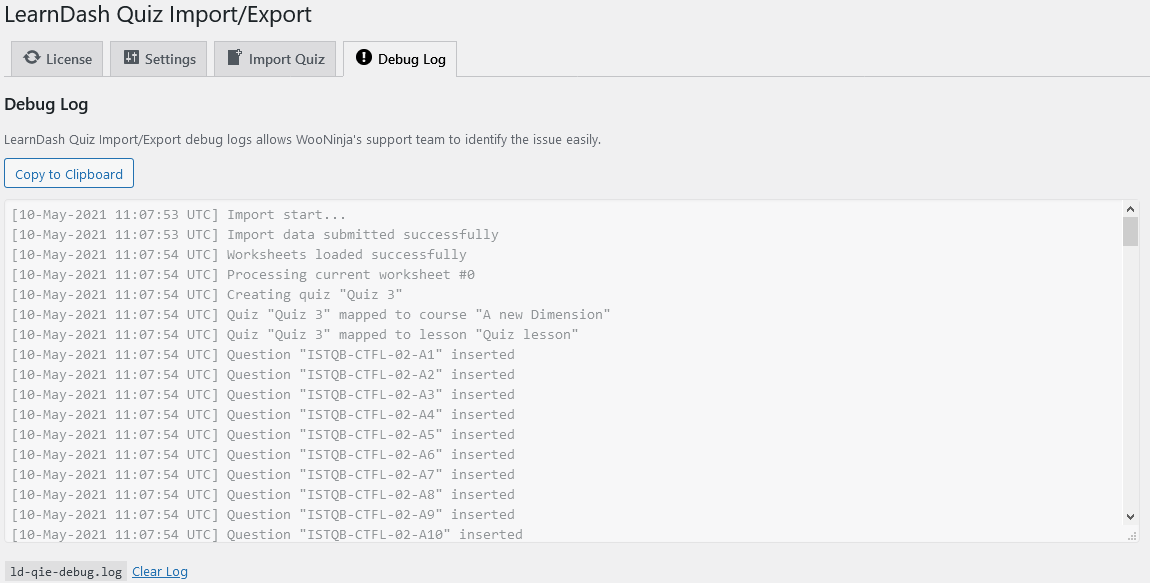How to Import a Single Quiz: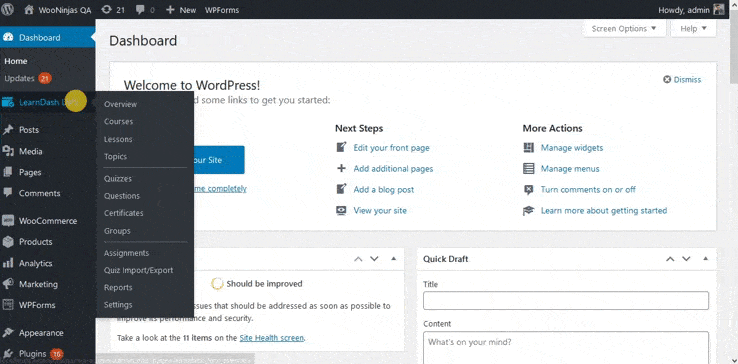How to Import Multiple Quizzes at a time: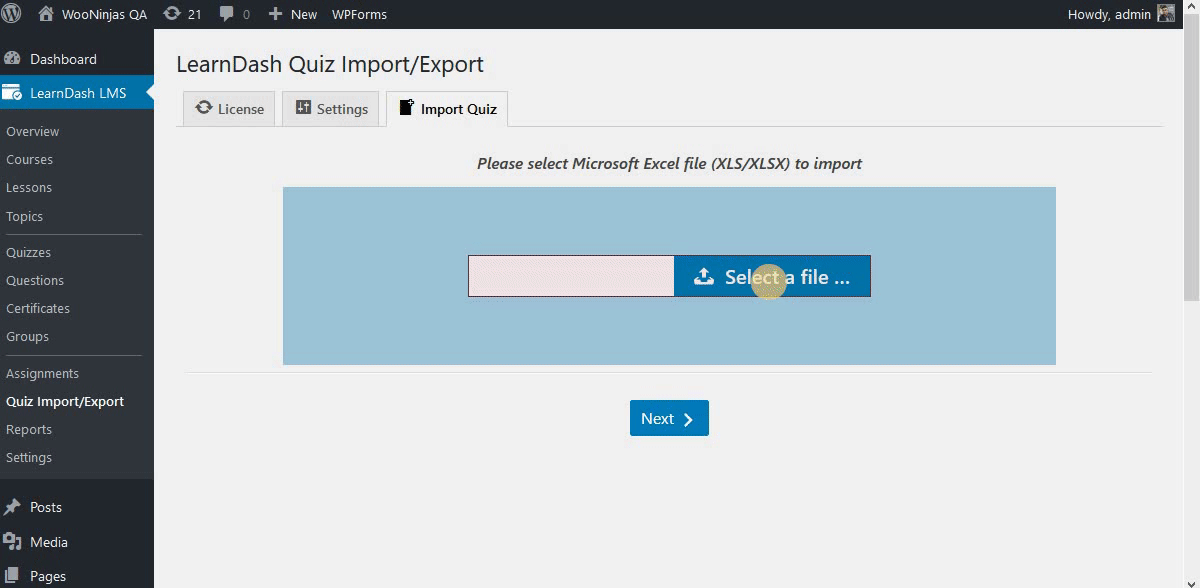# LearnDash Quiz Import/Export uses a standard template to import and export all LearnDash question types. This template should be filled in the given order to create your own templates for import/export. Downloading The Template:  The standard template can be found in the plugin folder: LearnDash-quiz-import-export > sample-import-files > Standard Template.xls The template contains the standard LearnDash question field names as column names. You will use the following columns which translate to LearnDash quiz questions:

## 1. Quiz Title

This column will hold the quiz title for the quiz to be imported.

# This represents the question title for a LearnDash quiz question.

Note: Same question titles are not allowed in a single quiz.

# After the individual answer and points column, you will find a column titled “Answer”. This column serves multiple purposes that vary according to the question type. Single choice → For a single choice answer, this column represents the correct answer from all the choices. Multiple choices

Multiple correct answers can be defined by placing a pipe sign (|) between them. For example, if there are 5 options (1,2,3,4,5) are available and only (2,3,5) are correct, then it can be defined like this 2|3|5.

Free choice

→ For a free choice answer, the column represents the single answer for this question type, as there can only be one answer. The answers can, however, be separated through line break. To add multiple answers for a free choice using this column, you need to add line breaks in the spreadsheet cell.

For.eg:

value1

value2

value3

(To add a line break in excel file use ALT + Enter when typing in the selected column cell)

Matrix Sorting Questions

For a matrix sort answer, the value inside these answer columns should be in

this format {criterion}{element}

Assessment

→ For an assessment answer, the answer column represents the single answer values for this question type. The answer should be typed in the following format:

{[ans1][ans2][ans3]}

Fill in the blank

→ For a closed (fill in the blank) answer, the column represents the value for the single correct answer for this question type. The answer should be typed in the following format:

This is a sample {cloze|5} question and It represents a sample cloze {type} question.

Note: A blank answer should be enclosed in {} and to enable a different number of points for each blank answer, the point has to be added after the pipe (|). If there is no separate point for each blank then there is no need to add the pipe (|) symbol.

Essay

→ For an essay answer, the column represents the answer submit method and the grading criteria. The following combination of values can be added to define the above values for this answer type.

Text submit, not graded and full points

Text submit, graded and full points

Text submit, not graded and no points

# Here you can enter a hint for the question-answer.

## 16. Material

Supporting material for the quiz, can be plain text or HTML.

## 17. Certificate awarded for

Required percentage to award a certificate.

## 18. Passing Percentage

Required percentage to pass a quiz.

## 19. Course

LearnDash course post ID to associate quiz with.

## 20. Lesson

LearnDash lesson post ID to associate quiz with.

## 21.Topic

LearnDash topic post ID to associate quiz with.

## 22. Certificate

LearnDash certificate post ID to assign to the quiz.

## 23. Shared Question

This column is optional and can be used only if the "Shared Quiz Questions" setting is enabled. To reuse a single question in multiple quizzes, set it to "1".

Note: To import multiple quizzes from a single excel file, duplicate the existing sheet and modify the data in it. You can add as many sheets as you want, but only one quiz is allowed per sheet.

# To use LearnDash Quiz Import/Export and Advanced Quizzes together, you’ll need to use a standard template to import and export all LearnDash question types and the advanced quizzes question types and settings. This template should be filled in the given order to successfully import/export quizzes that include the Advanced Quizzes question types and settings. Downloading The Template:  The advanced quizzes supported template can be found in the plugin folder: LearnDash-quiz-import-export > sample-import-files > Sample Template with Advance Quiz Support.xls The template contains the standard LearnDash question fields represented as columns with support for LearnDash Advanced Quizzes add-on features. On this template, the following columns should be filled to import information from the spreadsheet and translate data to LearnDash quiz questions:

## 1. Quiz Title

This column will hold the quiz title for the quiz to be imported.

# This represents the question title for a LearnDash quiz question.

Note: Same question titles are not allowed in a single quiz.

# These columns should be set according to the maximum number of answers present in any of the questions for the particular quiz import. If a quiz contains 13 answers for a particular question inside it, there should be 13 columns in the template. From: Answer 1 – Answer 13 Point 1 – Point 13 This column will contain all the answers which are available for a question. If a question has fewer answers than the maximum answer # column, then simply leave the unused columns empty. The point # column associates points with each answer. This column is used when the “different points for each answer” option is enabled. If your Question type is a jumbled sentence, the answer columns will be dependent on the number of variables your question contains. Answer 1 will contain the number of choices offered for the First variable of your question and Answer 2 column will have the choices offered for the second variable of the question respectively. If the Question type is Swipe Question this will contain the choices for the swipe questions. If your question type is Calculated Formula, this column will contain the Calculation formula as well as the values of the variables of the questions you want to offer. Eg. If our calculation formula for the question is ‘[x] + [y]’ and we want to offer value of x as 1 or 2 and the  value of y as 3 or 4, we will fill the column as  “ [x] + [y] | 1,2|3,4  ” Note: 1. For a matrix sort answer, the value inside these answer columns should be in this format {criterion}{element}.

After the individual answer and points column, you will find a column titled “Answer”. This column serves multiple purposes that vary according to the question type.

Single choice

→ For a single choice answer, this column represents the correct answer from all the choices.

Multiple choices

Multiple correct answers can be defined by placing a pipe sign (|) between them. For example if there are 5 options (1,2,3,4,5) are available and only (2,3,5) are correct, then it can be defined like this 2|3|5.

Free choice

→ For a free choice answer, the column represents the single answer for this question type, as there can only be one answer. The answers can, however, be separated through line break. To add multiple answers for a free choice using this column, you need to add line breaks in the spreadsheet cell.

For.eg:

value1

value2

value3

(To add a line break in excel file use ALT + Enter when typing in the selected column cell)

Matrix Sorting Questions

For a matrix sort answer, the value inside these answer columns should be in

this format {criterion}{element}

Assessment

→ For an assessment answer, the answer column represents the single answer values for this question type. The answer should be typed in the following format:

{[ans1][ans2][ans3]}

Fill In the Blank

→ For a closed (fill in the blank) answer, the column represents the value for the single correct answer for this question type. The answer should be typed in the following format:

This is a sample {cloze|5} question and It represents a sample cloze {type} question.

Note: A blank answer should be enclosed in {} and to enable a different number of points for each blank answer, the point has to be added after the pipe (|). If there is no separate point for each blank then there is no need to add the pipe (|) symbol.

Essay

→ For an essay answer, the column represents the answer submission method and the grading criteria. The following combination of values can be added to define the above values for this answer type.

Text submit, not graded and full points

Text submit, graded and full points

Text submit, not graded and no points

Jumbled Questions

→This column contains the correct answer to the question. It represents the correct answer(s) from all the choices. The pattern to indicate the correct choice is as follows:

The pipe (|) sign is used to separate answer numbers in a list when your question has more than 1 variable. For example, if a question has 5 different answers, the formatting would look as follows: |1|2|3|4|5. However, only the correct answer numbers should be included in this format (therefore numbers representing incorrect answers should be deleted). For example, if only answers 2,3, and 5 were correct you should fill this column as follows |2|3|5 and leave other spaces blank. If all answers are correct, then all answers should be listed.

|2|4|6 → This indicates that answer # 2,4 and 6 are correct for this multiple choice question and answer # 1,3 and 5 are not correct.

Swipe Questions

→It contains the correct answer to the question. It represents the correct answer(s) from all the choices. The pattern to indicate the correct choice is as follows:

The pipe (|) sign is used to separate answer numbers in a list if there are more than one correct accepted answers. For example, if a question has 5 different answers, the formatting would look as follows: |1|2|3|4|5. However, only the correct answer numbers should be included in this format (therefore incorrect answer should be deleted. For example, if only answers 2,3, and 5 were correct you should fill this column as follows |2|3|5. If all answers are correct, then all answers should be listed.

|2|4|6 → This indicates that answer # 2,4 and 6 are correct for this multiple choice question and answer # 1,3 and 5 are not correct.

Calculated Formula Questions

→If your question type is a calculated formula question then leave this column as empty.

# Here you can enter a hint for the question-answer.

## 18. Material

Supporting material for quiz, can be plain text or HTML.

## 19. Certificate awarded for

Required percentage to award a certificate.

## 20. Passing Percentage

Required percentage to pass a quiz.

## 21. Course

LearnDash course post ID to associate quiz with.

## 22. Lesson

LearnDash lesson post ID to associate quiz with.

## 23. Topic

LearnDash topic post ID to associate quiz with.

## 24. Certificate

LearnDash certificate post ID to assign to the quiz.

## 25. Shared Question

This column is optional and can be used only if the "Shared Quiz Questions" setting is enabled, set it to "1" to reuse a single question in multiple quizzes.

Note: To import multiple quizzes from a single excel file, duplicate the existing sheet, and modify the data in it. You can add as many sheets as you want, but only one quiz is allowed per sheet.